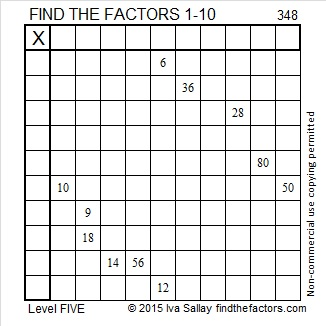# 348 and Level 5

Quick Prime/Composite Number Test: 348 is even and thus divisible by 2, so 348 is a composite number.Print the puzzles or type the factors on this excel file: 10 Factors 2015-01-05

• 348 is a composite number.
• Prime factorization: 348 = 2 x 2 x 3 x 29, which can be written 348 = (2^2) x 3 x 29
• The exponents in the prime factorization are 2, 1, and 1. Adding one to each and multiplying we get (2 + 1)(1 + 1)(1 + 1) = 3 x 2 x 2 = 12. Therefore 348 has exactly 12 factors.
• Factors of 348: 1, 2, 3, 4, 6, 12, 29, 58, 87, 116, 174, 348
• Factor pairs: 348 = 1 x 348, 2 x 174, 3 x 116, 4 x 87, 6 x 58, or 12 x 29
• Taking the factor pair with the largest square number factor, we get √348 = (√4)(√87) = 2√87 ≈ 18.655This site uses Akismet to reduce spam. Learn how your comment data is processed.